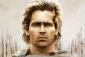# Arithmetic sequence

4 posts / 0 new
dan.and.yel
Arithmetic sequence

1/3, 1, 1/6, 2

is this an arithmetic sequence? i have seen it in a grade 10 book, thanks

Jhun VertNo it is not.

AlexanderArranged the numbers in increasing value: 1/6, 1/3, 1, 2. For this sequence to be an arithmetic sequence, the difference of any two terms (any term - preceding term) mus be equal. Let us check:

(a) 1/3 - 1/6 = 1/6
(b) 1 - 1/3 = 2/3
(c) 2 - 1 = 1

Since the difference of two adjacent terms are not equal, the given terms do not form into arithmetic sequence.

ケネス ケネスArithmetic sequence must be in increasing and deceasing value.

• Mathematics inside the configured delimiters is rendered by MathJax. The default math delimiters are $$...$$ and $...$ for displayed mathematics, and $...$ and $...$ for in-line mathematics.